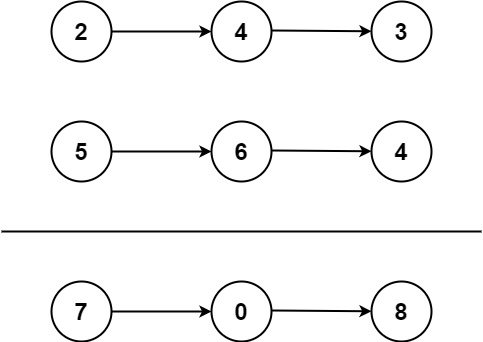• You are given two non-empty linked lists representing two non-negative integers. The digits are stored in reverse order, and each of their nodes contains a single digit. Add the two numbers and return the sum as a linked list.
• You may assume the two numbers do not contain any leading zero, except the number 0 itself.

### Example 1:Input: l1 = [2,4,3], l2 = [5,6,4]
Output: [7,0,8]
Explanation: 342 + 465 = 807.

### Example 2:

Input: l1 = , l2 = 
Output: 

### Example 3:

Input: l1 = [9,9,9,9,9,9,9], l2 = [9,9,9,9]
Output: [8,9,9,9,0,0,0,1]

### Constraints:

• The number of nodes in each linked list is in the range `[1, 100]`.
• `0 <= Node.val <= 9`
• It is guaranteed that the list represents a number that does not have leading zeros.

### Java Example

```class Solution { public ListNode addTwoNumbers(ListNode l1, ListNode l2) { ListNode ans = new ListNode(0); ListNode curr = ans; int carry = 0; while (l1 != null || l2 != null || carry > 0) { if (l1 != null) { carry += l1.val; l1 = l1.next; } if (l2 != null) { carry += l2.val; l2 = l2.next; } curr.next = new ListNode(carry % 10); carry /= 10; curr = curr.next; } return ans.next; } } // 2023 ```

### C++ Example

```class Solution { public: ListNode* addTwoNumbers(ListNode* l1, ListNode* l2) { ListNode ans(0); ListNode* curr = &ans; int carry = 0; while (l1 || l2 || carry) { if (l1 != nullptr) { carry += l1->val; l1 = l1->next; } if (l2 != nullptr) { carry += l2->val; l2 = l2->next; } curr->next = new ListNode(carry % 10); carry /= 10; curr = curr->next; } return ans.next; } }; // 2023 ```

### Complexity :

Time complexity: O(N);
Space Complexity: O(1);

I hope you like this Amazing Add Two Numbers article.🙂